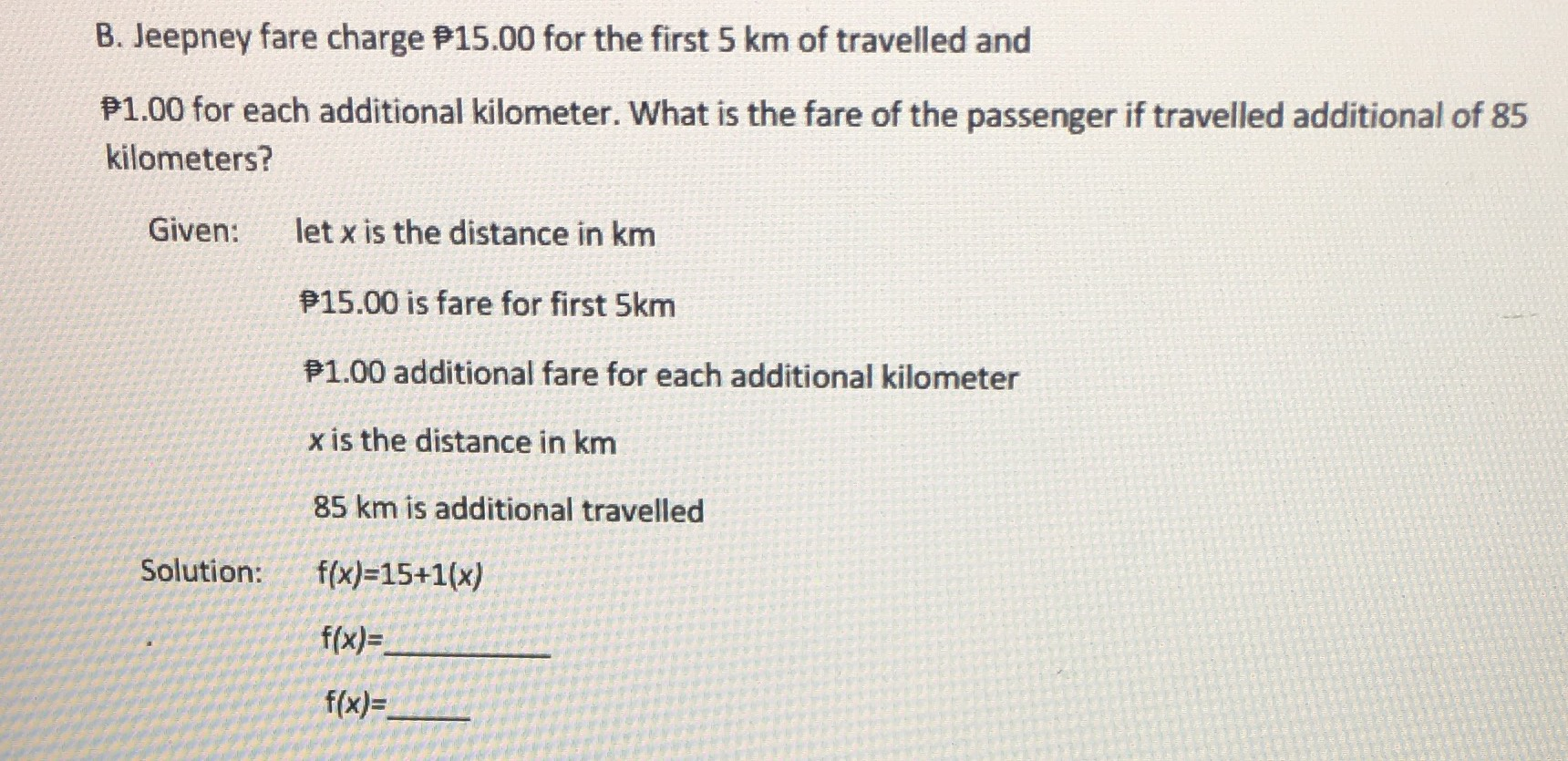### Still have math questions?

Algebra
QuestionB. Jeepney fare charge $$₹ 15.00$$ for the first $$5 km$$ of travelled and ₹ $$1.00$$ for each additional kilometer. What is the fare of the passenger if travelled additional of $$85$$ kilometers? Given: let $$x$$ is the distance in $$km$$ P15.00 is fare for first $$5 km$$ P1.00 additional fare for each additional kilometer

$$x$$ is the distance in $$km$$

$$85 km$$ is additional travelled

$$f ( x ) = 15 + 1 ( x )$$

$$f ( x ) =$$

$$f ( x ) =$$